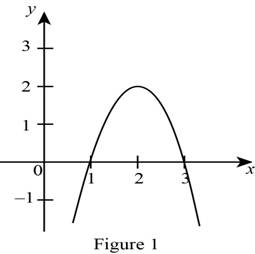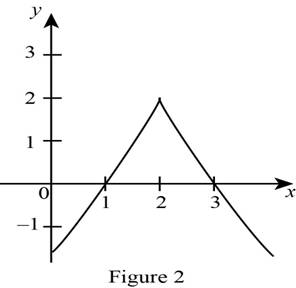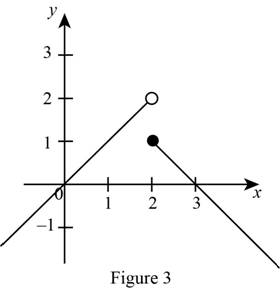# The graph of a function thatsatisfies the conditions that the graph has local maximum at 2 and is differentiable at 2.### Single Variable Calculus: Concepts...

4th Edition
James Stewart
Publisher: Cengage Learning
ISBN: 9781337687805### Single Variable Calculus: Concepts...

4th Edition
James Stewart
Publisher: Cengage Learning
ISBN: 9781337687805

#### Solutions

Chapter 4.2, Problem 11E

a)

To determine

## To sketch: The graph of a function thatsatisfies the conditions that the graph has local maximum at 2 and is differentiable at 2.

Expert Solution

### Explanation of Solution

Let the x be represented in the x-axis and the value of the function f(x) be represented in the y-axis.

Since an absolute maximum occurs at the point 2, choose the greatest point on x-axis. That is, consider the point on (2, 2).

The graph of a differentiable function must have a tangent at each point in its domain. it should be a smooth curve and cannot contain any break or cusps.

Since the graph is differentiable at 2, the derivative of f(2) exists.

Draw the graph of the function f in such a way that it satisfies the given conditions as shown below in Figure 1.From Figure 1, it is observed that the absolute maximum occurs at x = 2.

Also observe that the graph of the function is continuous and differentiable at x = 2.

b)

To determine

Expert Solution

### Explanation of Solution

Let the x be represented in the x-axis and the value of the function f(x) be represented in the y-axis.

Since the local maximum occurs at the point 2, choose the point 2 at any open interval of a domain. That is, consider the point on (2, 2).

Since the graph is not differentiable at 2 the derivative of f(2) does not exists.

Draw the graph of the function f in such a way that it satisfies the given conditions as shown below in Figure 2.From Figure 2 observe that the local maximum occurs at x = 2. And the curve is continuous.

Also, observe that the graph not differentiable at x = 2.

c)

To determine

Expert Solution

### Explanation of Solution

Let the x be represented in the x-axis and the value of the function f(x) be represented in the y-axis.

Since local maximum occurs at the point 2, choose the point 2 on the open interval of a domain. That is, consider the point on (2, 2).

A function is discontinuous at x = 2 if the graph of the function has break at that point.

Draw the graph of the function f in such a way that it satisfies the given conditions as shown below in Figure 3.From Figure 3 observe that the local maximum occurs at x = 2 and the function is not continuous at x = 2.

### Have a homework question?

Subscribe to bartleby learn! Ask subject matter experts 30 homework questions each month. Plus, you’ll have access to millions of step-by-step textbook answers!